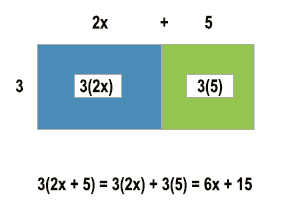# Teacher’s Lounge Blog

Learn more about teacher preparation, test tips, online learning, professional development, and a variety of other valuable teacher topics.

# Sample MTEL65 Middle School Math Open Response

May 14th, 2021 | Comments Off on Sample MTEL65 Middle School Math Open Response | Certification Prep, Math Certification, Teacher's Lounge Blog, Teaching Licenses

MA DESE is updating many MTEL exams over the coming year.  As of the end of May, the MTEL 47 Middle School Math exam will be replaced by the MTEL 65 Middle School Math exam.  This is a welcome change for many as many of the more difficult concepts, including calculus and discrete math, were removed for this new exam.  There will still be two open response questions on this MTEL exam and one is quite different than the previous exam. Here is a quick sample problem with a response.  For more information on preparing for this exam, please check out our full-length, MTEL prep comprehensive course with unlimited expert instructor support. (www.prepforward.com/mtel-massachusetts)

The following is a sample problem for Objective 14 on the new MTEL 65 exam.

Standard: The Massachusetts Mathematics Curriculum Framework for grade 7 provides the following content standard:
Expressions and Equations (7.EE)
1. Apply properties of operations to add, subtract, factor, and expand linear expressions with rational coefficients.

Problems

1. Solve for x:  3x – 7 = 2/3
2. Factor  x2 + 4x + 4
3. Simplify  6(-x + 0.5)

Assignment
Analyze standard 7.EE.1.  Identify related prerequisite concepts and skills.  Critique whether the problems listed above are aligned with the content standard. Provide your own problem with different representations to teach the standard. Explain your reasoning.

Here is a sample response for the above problem.

The 7th grade standard under Expressions and Equations involves an application of the properties of operations to add, subtract, factor, and expand linear expressions with rational coefficients. Therefore, a student must understand associative, commutative, and distributive properties as a prerequisite. Furthermore, as the coefficients are rational, a student must be comfortable with all the operations with rational numbers, including negatives, fractions, and decimals. Finally, students must understand how to identify if two expressions are equivalent.

Given Problems:

1. Solve for x:  3x – 7 = 2/3
2. Factor  x2 + 4x + 4
3. Simplify  6(-x + 0.5)

There were three sample problems given for teaching this standard. Unfortunately, the first sample problem does not fit under this standard as the problem asks the student to solve an equation, which is a concept that is taught later as this standard only deals with expressions. The second sample problem also does not fit under this standard as it is not a linear expression, but a quadratic expression. The third sample problem is an appropriate question to ask students under this standard.

In addition to problems similar to the third sample problem, one part of this standard is teaching students to use the distributive property to find equivalent expressions.  An area model will help students visualize this.  A sample is included below.

In this problem, a student would be asked to simplify 3(2x + 5).  Using the area model, they would be asked to find the area of each rectangle and then add them together to find the total area of both rectangles.